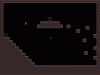# GMLCharacters Sudden impetuous

B

#### BinFanar

##### Guest
Presently, I am undertaking Gm2 course and hitherto this is the movement code:

Code:
``````/// movePlayerState()

// if player in the air
if (!place_meeting(x, y+1, Solid)) {
velV += accelG;

//Control player jump sprite
sprite_index = sPlayerJump;
image_speed = 0;

image_index = (velV > 0);// return true 1, 0; not an animation change image

// Control jump
if (upRelease && velV < -6) {
velV = -6;
}

} else {
velV = 0;

// Jump
if (up) {

velV = -16;

}

// player on ground
if (velH == 0 ) {
sprite_index = sPlayerIdle;
} else {
sprite_index = sPlayerWalk;
image_speed = .6;
}
}

//Check for collision by proffer 3 variables. Ex1

/*
if (right && !place_meeting(x+velX, y, Solid)) {
x += velX;
}

if (left && !place_meeting(x-velX, y, Solid)) {
x -= velX;
}

if (up && !place_meeting(x, y-velY, Solid)) {
y -= velY;
}

if (down && !place_meeting(x, y+velY, Solid)) {
y += velY;
}
*/

/* old movement code
if (right) {
velH = velX;
playerDir = 1;
}

if (left) {
velH = -velX;
playerDir = -1;
}
*/

// if either pressed
if (right || left) {
velH += (right-left)*playerAccel; // (right-left) 1 or -1
playerDir = right - left;

// Max speed limit
if (velH > velX) velH = velX; // accel right
if (velH < -velX) velH = -velX; //accel left
} else {
// Friction
checkFriction(playerAccel);
}

/* old Friction
if (!left && !right) {
velH = 0;
}
*/

// if character is moving, invert the sprite to the direction of the movement
if (velH != 0) {
image_xscale = sign(velH);
}

/* if (!up && !down) {
velV = 0;
}*/

// play landing sound

checkCollision(Solid);

x += velH;
y += velV;

// check for ledge grab
show_debug_message("yprevious = " + string(yprevious));
show_debug_message("yposition = "+ string(y));

var falling = y-yprevious > 0;// if falling +1 else 0
var noWall = !position_meeting(x+17 * image_xscale, yprevious, Solid);
var isWall = position_meeting(x+17 * image_xscale, y, Solid);

if (falling && noWall && isWall) {

velV = 0;
velH = 0;

// Move agains the ledge. sign(velV) could have been substituted for image_xscale
while(!place_meeting(x+image_xscale, y, Solid)){
x += image_xscale;
}

//Why y-1?, what is the position of x, y? is it 0,0
while (position_meeting(x+17*image_xscale, y-1, Solid)) {
y-=1;
}
sprite_index = sPlayerLedgeGrab;
playerState = ledgeGrabState;
}``````
I fully understand the preceding code. However, I wanted to contrive another code and I was trying to use isMoving to change the character's sprite direction. but whenever I press the left/right key, after gaining momentum, to switch to the opposite direction, the character moves with unremitting speed (see gif below). Why is it behaving in this manner? I am only changing the value of Image_xscale. it took me hours to suss out this abhorred fiendCode:
``````var isMoving = right - left;
var isJumping = up;

if (isMoving !=0) {
velH += isMoving * momentum;

if (velH > walkSp) velH = walkSp;
if (velH < -walkSp) velH = -walkSp;
} else {
checkFriction(momentum);
}

if (velH != 0) {
image_xscale = sign(velH);
}

if (isMoving == 0){
sprite_index = sPlayerIdle;
} else {
sprite_index = sPlayerWalk;
image_speed = 0.6;
}

x += velH;
y += velV;``````
Naughty Player:I shall be contented with your criticism and remarks.

#### Attachments

• 318.4 KB Views: 2

#### Simon Gust

##### Member
I remember having the same issues when I first made a platformer. It has something to do with pressing the opposite button in a certain I believe.
Anyway, could you show us, what the code inside checkFriction looks like?

EDIT: That said, I suggest a different approach to momentum.
Code:
``````var momentum = 0.5;

if (isMoving != 0)
{
if (isMoving > 0) velH = min(velH + momentum, walkSp);
else
if (isMoving < 0) velH = max(velH - momentum, -walkSp);
}
else
{
if (velH > 0) velH = max(velH - momentum, 0);
else
if (velH < 0) velH = min(velH + momentum, 0);
}``````
This approach never makes changes to the momentum, only to velH.
At the same time, it's preventing velH from going over walkSp while adding / subtracting momentum.

Last edited:
B

#### BinFanar

##### Guest
I remember having the same issues when I first made a platformer. It has something to do with pressing the opposite button in a certain I believe.
Anyway, could you show us, what the code inside checkFriction looks like?

EDIT: That said, I suggest a different approach to momentum.
Code:
``````var momentum = 0.5;

if (isMoving != 0)
{
if (isMoving > 0) velH = min(velH + momentum, walkSp);
else
if (isMoving < 0) velH = max(velH - momentum, -walkSp);
}
else
{
if (velH > 0) velH = max(velH - momentum, 0);
else
if (velH < 0) velH = min(velH + momentum, 0);
}``````
This approach never makes changes to the momentum, only to velH.
At the same time, it's preventing velH from going over walkSp while adding / subtracting momentum.
Thank you for responding. This is the friction code:

Code:
``````var momentum = argument0;

if (velH != 0) { // if character is moving
if (abs(velH)-momentum > 0) {
velH -= momentum * image_xscale;
} else {
velH = 0;
}

}``````